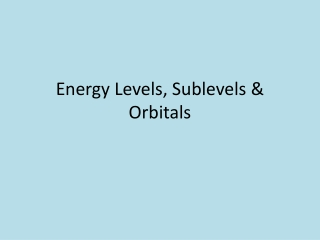DownloadDownload PresentationEnergy Levels, Sublevels & Orbitals

# Energy Levels, Sublevels & Orbitals

Télécharger la présentation## Energy Levels, Sublevels & Orbitals

- - - - - - - - - - - - - - - - - - - - - - - - - - - E N D - - - - - - - - - - - - - - - - - - - - - - - - - - -
##### Presentation Transcript

1. Energy Levels, Sublevels & Orbitals

2. Electron Review • Exact location unknown • Found outside of the nucleus in electron cloud • Found in energy levels • Energy levels closer to the nucleus have less energy than those farther from the nucleus • Electrons fill the energy levels closest to the nucleus first

3. Energy Levels • Total of 7 • 1st energy level is closest to nucleus • Contain sublevels • Four types • s (lowest energy) • p • d • f (highest energy)

4. Each energy level does not contain the same sublevels • As the distance from the nucleus increases • energy levels can hold more electrons • Therefore, they can have more sublevels

5. Energy Levels & Their Sublevels If you want to name a specific sublevel in an energy level, you write the energy level number followed by the sublevel. Example: 3rd energy level, d sublevel is written as 3d.

6. Each sublevel contains at least one orbital • Area of higher probability of finding electrons • Every orbital holds 2 electrons • Different sublevels have different shaped orbitals • s = spherical • p = dumbbell

7. Energy Levels and Electrons 2 2 + 6 = 8 2 + 6 + 10 = 18 2 + 6 +10 + 14 = 32 32 32

8. There is some overlap between energy sublevels • d sublevel has slightly more energy than the s sublevel of the next energy level • Example: • 3rd energy level d sublevel > 4th energy level s sublevel • means that electrons will fill a 4s sublevel before it fills a 3d sublevel

9. Aufbau Principle • states order of how electrons fill energy levels and sublevels • Always fill lowest energy levels and sublevels first